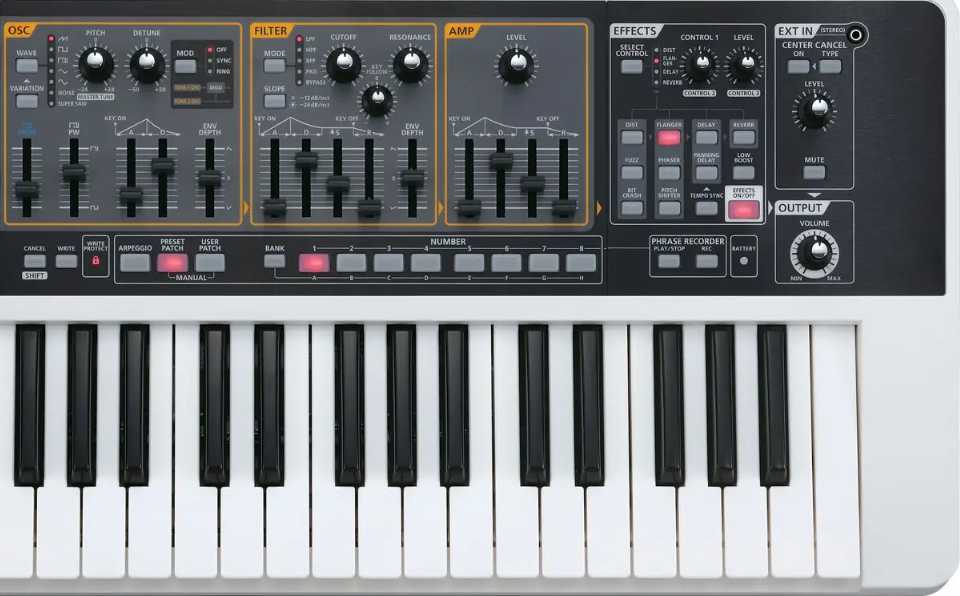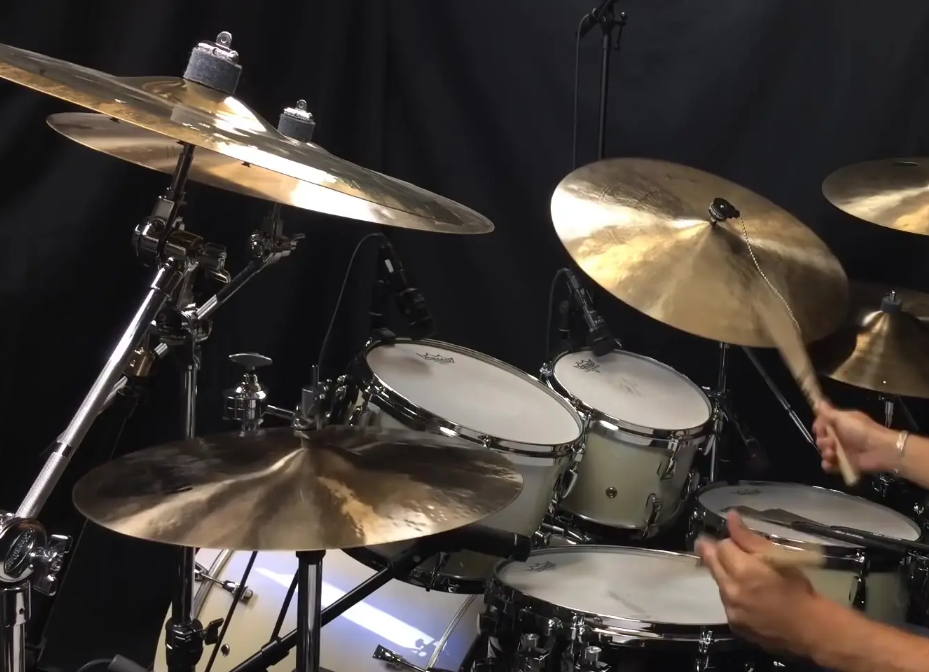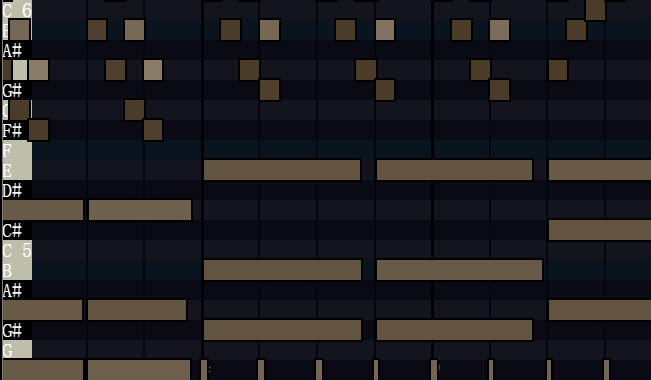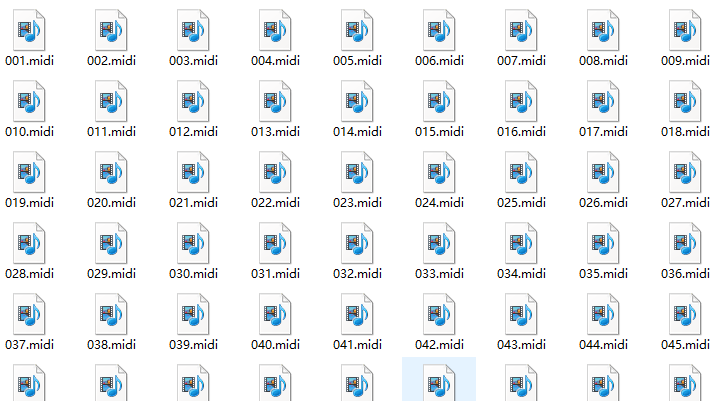# 老張說：快過年了，搞個AI作曲，用TensorFlow訓練midi檔案

## 一、老張需求：AI作曲營造新年氛圍

“嗯，你特別的煩人”。

“說事情！”## 二、midi格式：行動式音樂描述檔案

`AI`作曲，遵循“種瓜得瓜，種豆得豆”的原則：你給它訓練什麼風格的樣本，它最終就會生成什麼風格的音樂。```python import pretty_midi

# 載入樣本檔案

pm = pretty_midi.PrettyMIDI("jqm.midi")

# 迴圈樂器列表

for i, instrument in enumerate(pm.instruments): instrument_name = pretty_midi.program_to_instrument_name(instrument.program) print(i, instrument_name) # 輸出樂器名稱 ```

```Acoustic Grand Piano(原聲大鋼琴)、Glockenspiel(鋼片琴)、String Ensemble(絃樂合奏) 、 Muted Trumpet(悶音小號)、Trombone(長號)、 Electric Bass(電貝斯)、Acoustic Guitar(原聲吉他)、 Flute(長笛)、Acoustic Grand Piano(原聲大鋼琴)、 Harmonica(口琴)、Vibraphone(電顫琴)、Bagpipe(蘇格蘭風笛)、Marimba(馬林巴琴)……```

``` python

# 承接上個程式碼片段，假設選定了樂器instrument

for j, note in enumerate(instrument.notes): # 音高轉音符名稱 note_name = pretty_midi.note_number_to_name(note.pitch) info = ("%s:%.2f->%.2f")%(note_name, note.start, note.end) ```

Acoustic Grand Piano

F#3:1.99->2.04 F#2:1.98->2.06 E2:1.99->2.07 C2:1.98->2.08 F#3:2.48->2.53 F#2:2.48->2.56 F#3:2.98->3.03 F#2:2.98->3.06 ……

| 名稱 | pitch | start | end | velocity | | --- | --- | --- | --- | --- | | 示例 | 24 | 1.98 | 2.03 | 82 | | 解釋 | 音高(C1、C2) | 開始時間 | 結束時間 | 力度 | | 範圍 | 128個音高 | 單位為秒 | 單位為秒 | 最高100 |## 三、實戰：TensorFlow實現AI作曲`AI`作曲，聽起來很高階。其實跟文字生成、詩歌生成，沒有什麼區別。我之前講過很多相關的例子《NLP實戰：基於LSTM自動生成原創宋詞》《NLP實戰：基於GRU的自動對春聯》《NLP實戰：基於RNN的自動藏頭詩》。也講過很多關於NLP的知識點《NLP知識點：Tokenizer分詞器》《NLP知識點：文字資料的預處理》等。如果感興趣，大家可以先預習一下。不看也不要緊，後面我也會簡單描述，但深度肯定不如上面的專項介紹。

First Citizen: Before we proceed any further, hear me speak.

All: Speak, speak.

| 當前 | 下一個 | 經驗值 | | --- | --- | --- | | F | i | ☆ | | Fi | r | ☆ | | Fir | s | ☆ | | Firs | t | ☆☆ | | …… | …… | …… |

`F`後面大概率會出現`i`。如果現在是`Fi`，那麼它的後面該出現`r`了。這些，`AI`作為經驗記了下了。

`AI`分析過人類歷史上，出現過的所有語言之後。當它進行資料分析的時候，最終它會計算出：在人類的語言庫裡，冬天出現飄雪花的情況，要遠遠高於冬天飄落葉的情況。所以，它肯定也會告訴你那個空該填：雪花。

### 3.1 準備：構建資料集

```Note(start=1.988633, end=2.035121, pitch=54, velocity=82), Note(start=1.983468, end=2.060947, pitch=42, velocity=78), Note(start=2.479335, end=2.525823, pitch=54, velocity=82)……```

```Note(duration=0.16, step=0.00, pitch=54), Note(duration=0.56, step=0.31, pitch=53), Note(duration=0.26, step=0.22, pitch=24), ……```

`duration`表示演奏時長，這個音符會響多久，它等於`end-start`

`step`表示步長，本音符距離上一個出現的時間間隔，它等於`start2-start1`

``` python import pretty_midi import tensorflow as tf

midi_inputs = [] # 存放所有的音符 filenames = tf.io.gfile.glob("datasets/*.midi")

# 迴圈所有midi檔案

for f in filenames: pm = pretty_midi.PrettyMIDI(f) # 載入一個檔案 instruments = pm.instruments # 獲取樂器 instrument = instruments # 取第一個樂器，此處是原聲大鋼琴 notes = instrument.notes # 獲取樂器的演奏資料 # 以開始時間start做個排序。因為預設是依照end排序 sorted_notes = sorted(notes, key=lambda note: note.start) prev_start = sorted_notes.start # 迴圈各項指標，取出前後關聯項 for note in sorted_notes: step = note.start - prev_start # 此音符與上一個距離 duration = note.end - note.start # 此音符的演奏時長 prev_start = note.start # 此音符開始時間作為最新 # 指標項：[音高（音符），同前者的間隔，自身演奏的間隔] midi_inputs.append([note.pitch, step, duration]) ````` 上面的操作，是把所有的```MIDI`檔案，依照預處理的規則，全部處理成`[pitch, step, duration]`格式，然後存放到`midi_inputs`陣列中。

``` python seq_length = 24 # 輸入序列長度 vocab_size = 128 # 分類數量

# 將序列拆分為輸入和輸出標籤對

def split_labels(sequences): inputs = sequences[:-1] # 去掉最後一項最為輸入 # 將音高除以128，便於 inputs_x = inputs/[vocab_size,1.0,1.0] y = sequences[-1] # 擷取最後一項作為輸出 labels = {"pitch":y, "step":y,"duration":y} return inputs_x, labels

# 搞成tensor，便於流操作，比如notes_ds.window

notes_ds = tf.data.Dataset.from_tensor_slices(midi_inputs) cut_seq_length = seq_length+1 # 擷取的長度，因為要拆分為輸入+輸出，因此+1

# 每次滑動一個數據，每次擷取cut_seq_length個長度

windows = notes_ds.window(cut_seq_length, shift=1, stride=1,drop_remainder=True) flatten = lambda x: x.batch(cut_seq_length, drop_remainder=True) sequences = windows.flat_map(flatten)

# 將25，拆分為24+1。24是輸入，1是預測。進行訓練

seq_ds = sequences.map(split_labels, num_parallel_calls=tf.data.AUTOTUNE) buffer_size = len(midi_inputs) - seq_length

# 拆分批次，快取等優化

train_ds = (seq_ds.shuffle(buffer_size) .batch(64, drop_remainder=True) .cache().prefetch(tf.data.experimental.AUTOTUNE)) ```

### 3.2 訓練：構建神經網路結構

``` python input_shape = (seq_length, 3) # 輸入形狀 inputs = tf.keras.Input(input_shape) x = tf.keras.layers.LSTM(128)(inputs)

# 輸出形狀

outputs = { 'pitch': tf.keras.layers.Dense(128, name='pitch')(x), 'step': tf.keras.layers.Dense(1, name='step')(x), 'duration': tf.keras.layers.Dense(1, name='duration')(x), } model = tf.keras.Model(inputs, outputs) ````` 上面程式碼我們定義了輸入和輸出的格式，然後中間加了個```LSTM`層。

|Layer (type) | Output Shape | Param | Connected to| | --- | --- | --- | --- | | input (InputLayer) | [(None, 24, 3)] | 0 | [] | | lstm (LSTM) | (None, 128) | 67584 | ['input'] | | duration (Dense) | (None, 1) | 129 | ['lstm'] | | pitch (Dense) | (None, 128) | 16512 | ['lstm'] | | step (Dense) | (None, 1) | 129 | ['lstm'] | | Total params: 84,354 | | |

``` python checkpoint_path = 'model/model.ckpt' # 模型存放路徑 model.compile( # 配置引數 loss=loss, loss_weights={'pitch': 0.05,'step': 1.0,'duration':1.0}, optimizer=tf.keras.optimizers.Adam(learning_rate=0.01), )

# 模型儲存引數

cp_callback = tf.keras.callbacks.ModelCheckpoint( filepath=checkpoint_path ,save_weights_only=True, save_best_only=True)

# 啟動訓練，訓練50個週期

model.fit(train_ds, validation_data=train_ds , epochs=50,callbacks=[cp_callback]) ````` 訓練完成之後，會將模型儲存在```'model/model.ckpt'`目錄下。而且，我們設定了只儲存最優的一個模型`save_best_only=True`。

``` python

# 從音符庫中隨機拿出24個音符，當然你也可以自己編

sample_notes = random.sample(midi_inputs, 24) num_predictions = 600 # 預測後面600個

# 迴圈600次，每次取最新的24個

for i in range(num_predictions): # 拿出最後24個 n_notes = sample_notes[-seq_length:] # 主要給音高做一個128分類歸一化 notes = [] for input in n_notes: notes.append([input/vocab_size,input,input]) # 將24個音符交給模型預測 predictions = model.predict([notes]) # 取出預測結果 pitch_logits = predictions['pitch'] pitch = tf.random.categorical(pitch_logits, num_samples=1) step = predictions['step'] duration = predictions['duration'] pitch, step, duration = int(pitch), float(step), float(duration) # 將預測值新增到音符陣列中，進行下一次迴圈 sample_notes.append([pitch, step, duration]) ````` 其實，關鍵程式碼就一句```predictions = model.predict([notes])`。根據`24`個音符，預測出來下一個音符的`pitch`、`step`和`duration`。其他的，都是輔助操作。

`24`個生成`1`個後，變成了`25`個。然後再取這`25`箇中的最後`24`個，繼續生成下一個。迴圈`600`次，最後生成了`624`個音符。列印一下：

```[[48, 0.001302083333371229, 0.010416666666628771], [65, 0.11979166666674246, 0.08463541666651508] …… [72, 0.03634712100028992, 0.023365378379821777], [41, 0.04531348496675491, 0.011086761951446533]]```

``` python

# 復原midi資料

prev_start = 0 midi_notes = [] for m in sample_notes: pitch, step, duration = m start = prev_start + step end = start + duration prev_start = start midi_notes.append([pitch, start, end]) ```

```[[48, 0.001302083333371229, 0.01171875], [65, 0.12109375000011369, 0.20572916666662877], …… [72, 32.04372873653976, 32.067094114919584], [41, 32.08904222150652, 32.100128983457964]]```

``` python

# 寫入midi檔案

pm = pretty_midi.PrettyMIDI() instrument = pretty_midi.Instrument( program=pretty_midi.instrument_name_to_program("Acoustic Grand Piano")) for n in midi_notes: note = pretty_midi.Note(velocity=100,pitch=n,start=n,end=n) instrument.notes.append(note) pm.instruments.append(instrument) pm.write("out.midi") ```

`MIDI`檔案有`5`個必需的要素。其中，樂器我們設定為`"Acoustic Grand Piano"`原聲大鋼琴。`velocity`沒有參與訓練，但也需要，我們設為固定值`100`。其他的`3`個引數，都是`AI`生成的，依次代入。最後，把結果生成到`out.midi`檔案中。「其他文章」